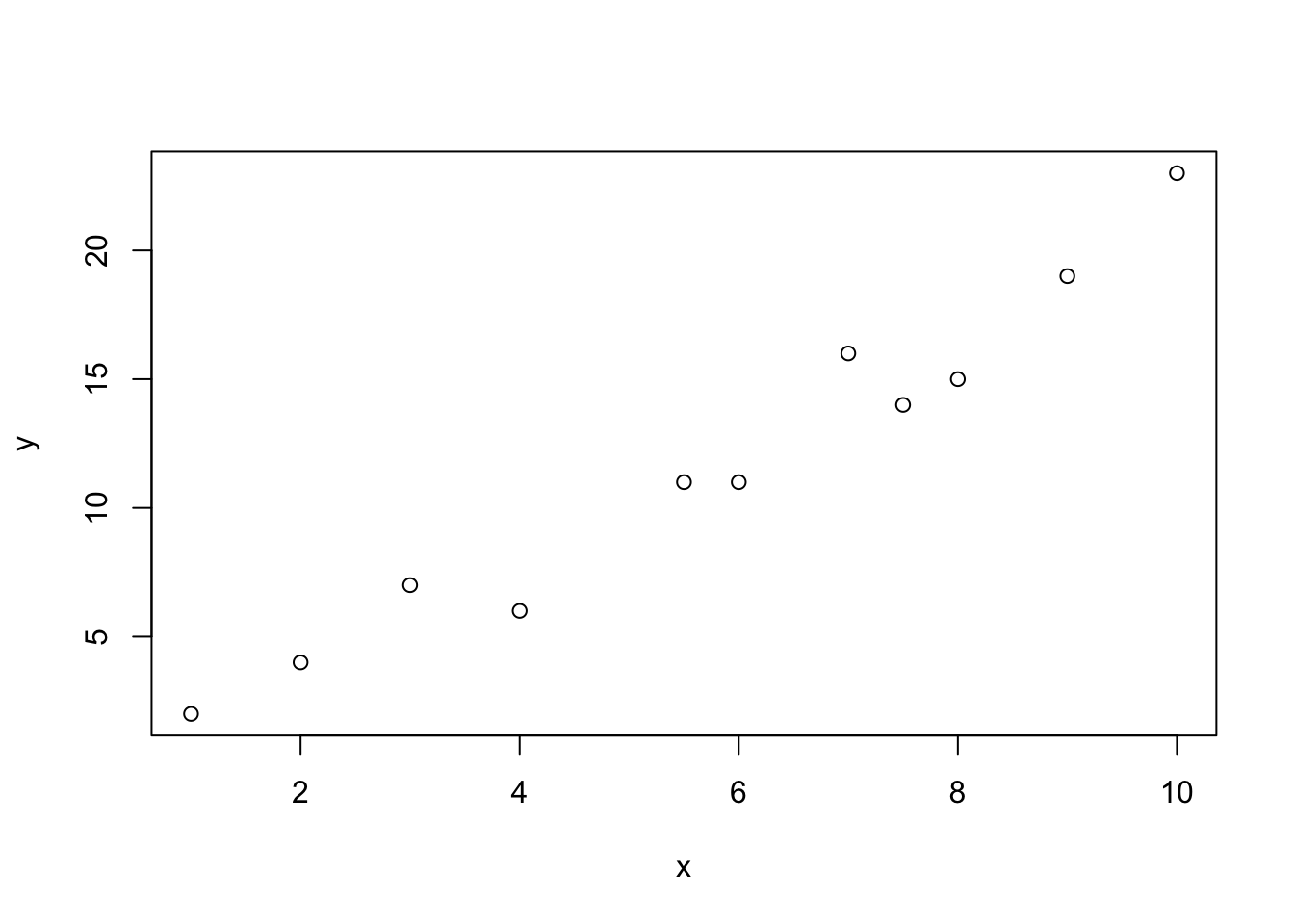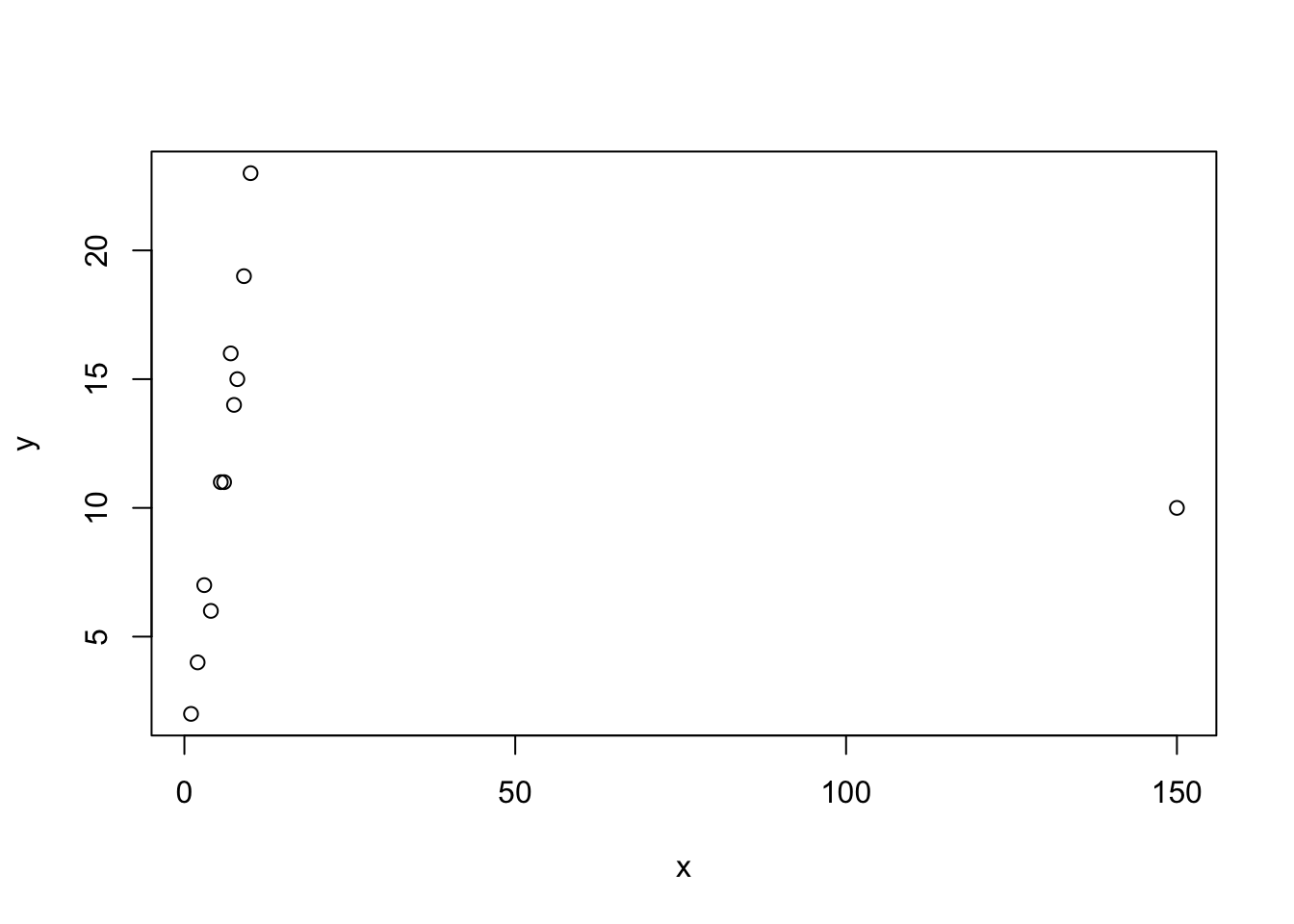### Part 1: Pearson’s coefficient vs Spearman’s coefficient

As we discussed, there are two widely used correlation coefficients, a Pearson’s one and a Spearman’s one. Since the latter is a measure of the rank correlation, it is usually used for variables in an ordinal scale. However, it can be helpful for quantitative variables as well because it is robust (not sensitive to outliers).

Consider two variables: x and y.

x <- c(1, 2, 6, 8, 9, 7, 7.5, 10, 3, 4, 5.5)
y <- c(2, 4, 11, 15, 19, 16, 14, 23, 7, 6, 11)

Let’s plot a simple scatterplot first:

plot(x, y)As we can see, although there are only few points, variables x and y seem to be positively associated (as x increases, y increases). We can even say that this association is pretty strong. Let’s calculate two correlation coefficients and test their statistical significance.

# Pearson's coefficient
cor.test(x, y)
##
##  Pearson's product-moment correlation
##
## data:  x and y
## t = 13.862, df = 9, p-value = 2.234e-07
## alternative hypothesis: true correlation is not equal to 0
## 95 percent confidence interval:
##  0.9124984 0.9942928
## sample estimates:
##       cor
## 0.9773737

What can we see in the output? The correlation coefficient itself is cor and here it is 0.977. So, we can conclude that the association between x and y is positive and very strong (the coefficient is approximately 1). Is it statistically significant at the 5% level of significance? Let us see.

$$H_0: corr(x, y) = 0 \text{ (no linear association between } x \text{ and } y )$$

This null hypothesis should be rejected at the 5% significance level since p-value < 0.05. So, variables x and y are associated.

# Spearman's coefficient
cor.test(x, y, method = 'spearman')
## Warning in cor.test.default(x, y, method = "spearman"): Cannot compute
## exact p-value with ties
##
##  Spearman's rank correlation rho
##
## data:  x and y
## S = 8.5188, p-value = 2.449e-06
## alternative hypothesis: true rho is not equal to 0
## sample estimates:
##       rho
## 0.9612781

Here we also get a very high positive coefficient (0.96). Now let us add an outlier, a non-typical observation to our data, a point (150, 10).

x <- c(1, 2, 6, 8, 9, 7, 7.5, 10, 3, 4, 5.5, 150)
y <- c(2, 4, 11, 15, 19, 16, 14, 23, 7, 6, 11, 10)
plot(x, y)It seems that this point can spoil everything! We can calculate correlation coefficient for updated variables:

cor.test(x, y)
##
##  Pearson's product-moment correlation
##
## data:  x and y
## t = -0.033164, df = 10, p-value = 0.9742
## alternative hypothesis: true correlation is not equal to 0
## 95 percent confidence interval:
##  -0.5808924  0.5668262
## sample estimates:
##         cor
## -0.01048683

A Pearson’s correlation coefficient has broken down! Now it is negative, very small by absolute value and, what is more, insignificant! This coefficient is very sensitive to outliers, so here it “reacts” on a non-typical point in a very dramatic way. Now let’s look at a Spearman’s coefficient:

cor.test(x, y, method = 'spearman')
## Warning in cor.test.default(x, y, method = "spearman"): Cannot compute
## exact p-value with ties
##
##  Spearman's rank correlation rho
##
## data:  x and y
## S = 64.613, p-value = 0.003127
## alternative hypothesis: true rho is not equal to 0
## sample estimates:
##       rho
## 0.7740817

Magic! This coefficient has not undergone serious changes, it is still positive and high. Besides, it is significant at the 5% significance level. So, with the help of this illustration we made sure that a Spearman’s correlation coefficient is more robust than Pearson’s one.

cor.test(x, y, method = 'kendall')
## Warning in cor.test.default(x, y, method = "kendall"): Cannot compute exact
## p-value with ties
##
##  Kendall's rank correlation tau
##
## data:  x and y
## z = 3.093, p-value = 0.001981
## alternative hypothesis: true tau is not equal to 0
## sample estimates:
##       tau
## 0.6870429

### Part 2: real data

Data description

Two hundred observations were randomly sampled from the High School and Beyond survey, a survey conducted on high school seniors by the National Center of Education Statistics. Source: UCLA Academic Technology Services.

Variables

• id: Student ID.
• gender: Student’s gender, with levels female and male.
• race: Student’s race, with levels african american, asian, hispanic, and white.
• ses: Socio economic status of student’s family, with levels low, middle, and high.
• schtyp: Type of school, with levels public and private.
• prog: Type of program, with levels general, academic, and vocational.
• read: Standardized reading score.
• write: Standardized writing score.
• math: Standardized math score.
• science: Standardized science score.
• socst: Standardized social studies score.

educ <- read.csv("education.csv")

And load tidyverse package and install GGally package that is a useful extension of ggplot2:

library(tidyverse)
library(GGally)

Now let us choose variables that correspond to abilities (read and write) and scores for subjects (math, science, socst).

scores <- educ %>% select(read, write, math, science, socst)

Let’s create a basic scatterplot matrix, a graph that includes several scatterplots, one for each pair of variables.

pairs(scores)`